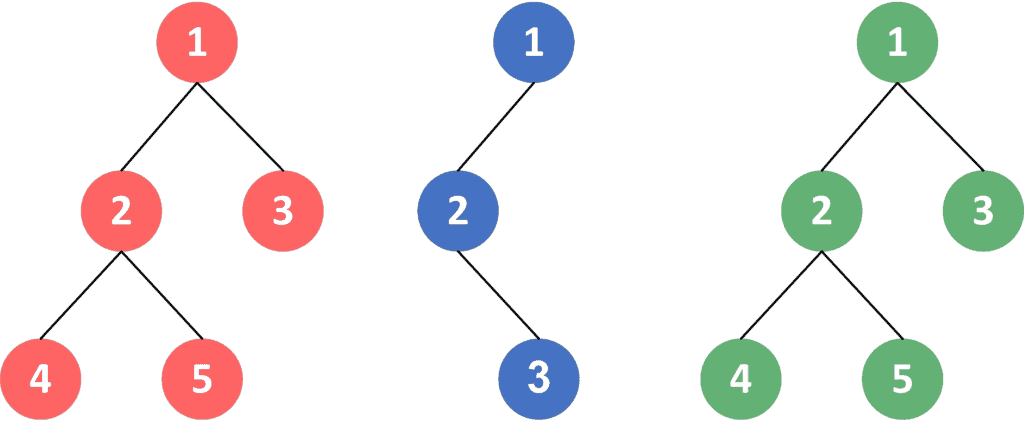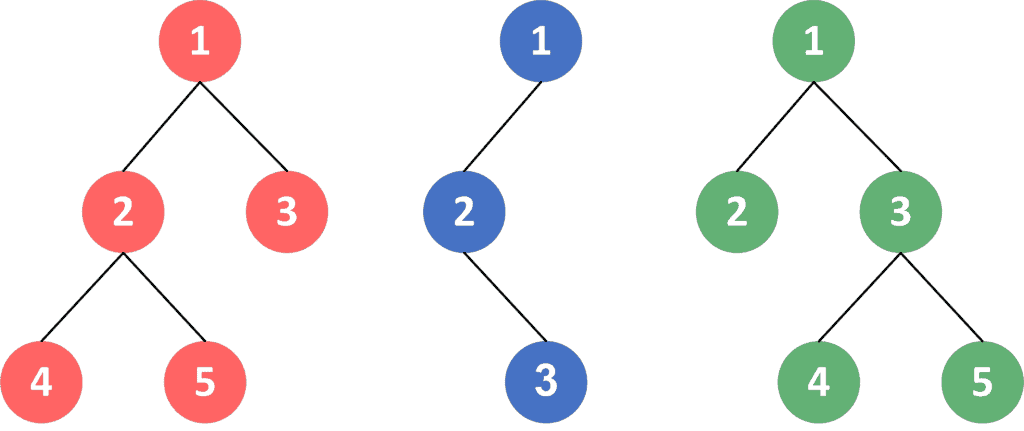## 1. Overview

In this article, we’ll discuss how to build a hash function for a tree structure.

First, we’ll define the problem and how we can judge whether two tree structures are different or not. Then, we’ll give an example to explain it. Finally, we’ll present an approach to solving this problem and give an extra follow-up idea to customize our hash function under certain constraints.

## 2. Defining the Problem

We have a tree structure ofnodes andedges. We want to get a hash code that represents the given tree structure. This can be used to compare any two tree structures in constant time.

Recall that a tree is a connected graph ofnodes andedges, such that there are no self-loops and no two edges connect the same pair of nodes.

Let’s take a look at an example for a better understanding.

Given the following three tree structures:After calculating the hash values for these trees, we might end up with values,, andthat represent the hashes for red, blue, and green trees, respectively.

As we can see, both the red and green trees have the same hash value because they look exactly the same. Meanwhile, the blue one has a different one because its shape is different.

## 3. Hash Tree Approach

The main idea in this approach is to build the hash of each node depending on the hash values of its children. When we get the hash of the root node, it’ll represent the hash of the whole tree.

We’ll dive into the given tree using DFS traversal. The moment we reach a leaf node, we return the hash of that single node as zero.

Then, when we go back to the DFS traversal, we can get the hash of the current node by using any hash algorithm on the sequence of hash values of its children. In our approach, we’ll use this formula to get the hash of a sequenceof length:Whereandare pre-defined constant values. We can change these values to get a different hash value.

Finally, when we get the hash of the root node, it’ll represent the hash of the whole tree. The reason is that to build the hash of each node in the tree, we used the hash values of all the nodes in its subtree.

### 3.1. Algorithm

Let’s take a look at the implementation of the algorithm:Initially, we define thefunction that will return the hash of the subtree that’s rooted at. The function will have one parameter,, which will represent the root of the subtree we want to hash.

First, we declare, which will store the hash of the current subtree that’s rooted at. Second, we iterate over the children of the current node and get the hash value of each one of them.

Third, we use the children’s hashes to get the hash value of the subtree, using the formula:Finally, thewill return the hash of the whole tree that is rooted at.

### 3.2. Complexity

The time complexity of this algorithm is, whereis the number of nodes in the given tree. The reason for this complexity is the same as DFS traversal complexity because we iterate over each node and each edge in the given tree only once.

## 4. Follow-Up

Let’s say we’re given a tree structure and we want to get its hash, but the order of the children doesn’t matter. Let’s take a look at an example:As we can see, if we swap the order of nodesandin the red tree, it becomes equal to the green one. So, the hash of the red and green trees should be the same. On the other hand, the blue one is not equal to either of the other trees, because there is no such sequence of operations of changing the order of children in the blue tree that can make it equal to either of the other two.

In this approach, we’ll use the same idea as the previous approach, but we’ll sort the hash values of children of each node before we get the hash of the current node since the order of the children doesn’t matter.

We’ll use DFS traversal as we did in the previous approach to get hash values of the current node’s children. However, before applying any hash algorithm on the sequence of children’s hash, we’ll sort that sequence to ignore the initial position of each child. Next, we’ll apply any hash algorithm on the sorted sequence to get the hash of the current node regardless of its children’s order.

Finally, the hash of the given tree will be the hash of the root of that tree.

### 4.1. Algorithm

Let’s take a look at the implementation of the algorithm:Initially, we define the samefunction as the previous approach that will return the hash of the subtree that’s rooted at. The function will have one parameter,, that will represent the root of the subtree we want to hash.

First, we declare an empty list calledthat will store the hash values of the current node’s children. Second, we iterate over the children of the current node and get the hash value of each of them and add it to thelist.

Third, we sort thelist to ignore the initial position of the current node’s children. Then, we get that hash of the sequence of the children’s hash using any hash algorithm. We used this formula to get the hash of the current node depending on the hash of its children:Finally,will return the hash of the whole tree that is rooted at.

### 4.2. Complexity

The complexity of this algorithm is, whereis the number of nodes in the given tree. The reason for this complexity is that we iterate over each node and each edge of the tree only once.

However, for each node, we sort its children’s hash in increasing order because the initial order of the children doesn’t matter. This operation has a complexity of.

Note that each node is a child of one node. Thus, it will be used for sorting only once.

## 5. Conclusion

In this article, we presented how to build a hash function for a tree structure.

First, we provided an example to explain the problem. Second, we explored an approach for solving it. Then, we gave an extra follow-up to get the hash of the tree structure regardless of the children’s order.

Finally, we walked through the implementations and explained the time complexity for each algorithm.